JEE  >  Mole Concept and Basic Concepts

# Mole Concept and Basic Concepts Notes | Study Additional Documents and Tests for JEE - JEE

## Document Description: Mole Concept and Basic Concepts for JEE 2022 is part of Documents for Additional Documents and Tests for JEE preparation. The notes and questions for Mole Concept and Basic Concepts have been prepared according to the JEE exam syllabus. Information about Mole Concept and Basic Concepts covers topics like and Mole Concept and Basic Concepts Example, for JEE 2022 Exam. Find important definitions, questions, notes, meanings, examples, exercises and tests below for Mole Concept and Basic Concepts.

Introduction of Mole Concept and Basic Concepts in English is available as part of our Additional Documents and Tests for JEE for JEE & Mole Concept and Basic Concepts in Hindi for Additional Documents and Tests for JEE course. Download more important topics related with Documents, notes, lectures and mock test series for JEE Exam by signing up for free. JEE: Mole Concept and Basic Concepts Notes | Study Additional Documents and Tests for JEE - JEE
 1 Crore+ students have signed up on EduRev. Have you?

MOLE CONCEPT
Definition of mole: One mole is a collection of those many entities as there are number of atoms exactly in 12 gm of C - 12 isotope.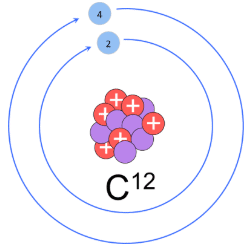Fig. C-12 isotope

Or 1 mole = collection of 6.02 × 1023 species
6.02 × 1023 = NA = Avogadro's No.
1 mole of atoms is also termed as 1 gm-atom, 1 mole of ions is termed as 1 gm-ion and 1 mole of molecule termed as 1 gm-molecule.

Methods of Calculations of mole:
(a) If no. of some species is given, then no. of moles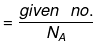(b) If weight of a given species is given, then no of moles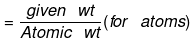or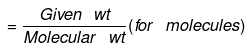(c) If volume of a gas is given along with its temperature (T) and pressure (P)

use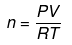where R = 0.0821 liter-atm/mol-K (when P is in atmosphere and V is in litre.)
1 mole of any gas at STP (0°C & 1 bar) occupies 22.7 litre.
1 mole of any gas at STP (0°C & 1 atm) occupies 22.4 litre.
Atom:  Atom is smallest particle which can not be divided into its constituents.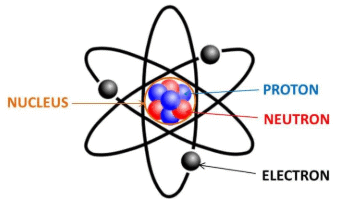Fig. Structure of atom

Atomic weight: It is the weight of an atom relative to one twelfth of weight of 1 atom of C-12 isotope.

Relationship between gram and amu:
⇒ 1 amu = 1/12 wt of one C - 12 atom
⇒ for C, 1 mole C = 12 gm = 6.023 × 1023 atoms
⇒ wt. of 6.023 × 1023 atoms = 12 gm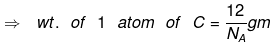(NA → Avogadro's number = 6.23 × 1023)
⇒ 1 amu = 1/12 wt of one C - 12 atom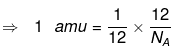⇒ 1 amu = 1/NA g
So, 1 amu = 1.66 x 10-24
= 1.66 x 10-27 kg .

Elemental Analysis:

For 'n' mole of a compound (C3H7O2)

Moles of C = 3n

Moles of H = 7n

Moles of O = 2n

Example. Find the weight of water present in 1.61 g of Na2SO4. 10H2O
Solution. Moles of Na2SO4.10H2O =  0.005 moles
Moles of water = 10 × moles of Na2SO4. 10H2O = 10 × 0.05 = 0.05
wt. of water = 0.5 × 18 = 0.9 gm

Average atomic weight:
= % of isotope x molar mass of isotope.
The % obtained by above expression (used in above expression) is by number (i.e. its a mole%).

Molecular weight:
It is the sum of the atomic weight of all the constituent atoms.
(a) Average molecular weight= where ni = no. of moles of any compound and
mi = molecular mass of any compound.
Shortcut for % determination if average atomic weight is given for X having isotopes XA & XB.
Try working out of such a shortcut for XA, XB, X

EMPIRICAL FORMULA and MOLECULAR FORMULA
Empirical formula: Formula depicting constituent atom in their simplest ratio.
Molecular formula: Formula depicting an actual number of atoms in one molecule of the compound.
Relation between the two: Molecular formula= Empirical formula × n
Check out the importance of each step involved in calculations of empirical formula.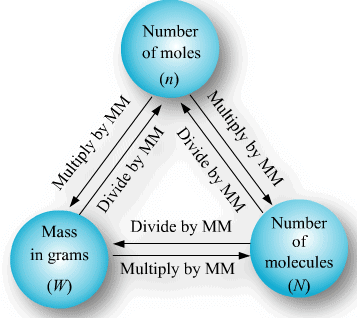Fig. Mole Concept

Example. A molecule of a compound has 13 carbon atoms, 12 hydrogen atom, 3 oxygen atoms and 3.02 × 10-23 gm of other element. Find the molecular wt. of compound.
Solution. Wt. of the 1 molecule of a compound = 13 × 12 × 3 × 3.02 × 10-23
= 234.18 / NA = 234 amu.

Density:
(a) Absolute density
(b) Relative density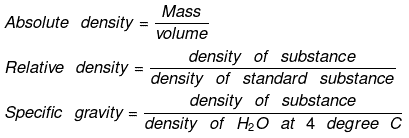Vapor density: It is defined only for gas.
It is a density of gas with respect to H2 gas at same temp & pressure.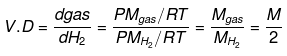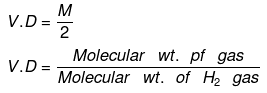Density of Cl2 gas with respect to O2 gas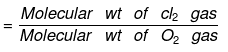The document Mole Concept and Basic Concepts Notes | Study Additional Documents and Tests for JEE - JEE is a part of the JEE Course Additional Documents and Tests for JEE.
All you need of JEE at this link: JEE

## Additional Documents and Tests for JEE

11 videos|159 docs|14 tests
 Use Code STAYHOME200 and get INR 200 additional OFF

## Additional Documents and Tests for JEE

11 videos|159 docs|14 tests

Track your progress, build streaks, highlight & save important lessons and more!

,

,

,

,

,

,

,

,

,

,

,

,

,

,

,

,

,

,

,

,

,

;